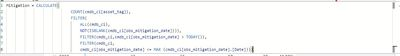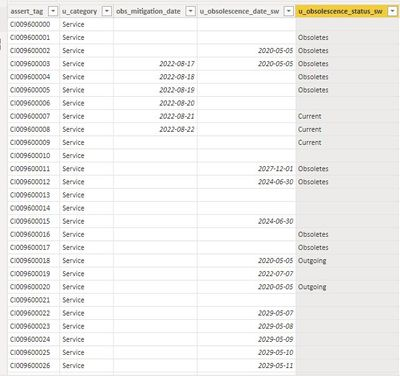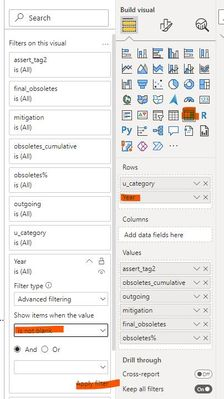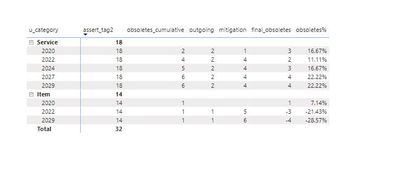cancel
Showing results for
Did you mean:Regular Visitor

## Cumulative Count in a Matrix with Dates

Hi

I need to display some data, cumulative in a matrix with future dates, however when i build the matrix the data is automatically segragated by year, as the print below.The total in the end is correct, an it basically shows the data in the way that I want, but i do need it in a yearly format, like the exemple below:For the culumns I used the following meaures syntax but still not working.Is there anythig that I missing or such parameters are not possible??

Data Details:
the table is cmdb_ci and I do have a dim_dates table.obsoletes_cumulative = count of rows where u_obsescence_status_sw = "Obsoletes",

outgoing = count of rows where u_obsescence_status_sw = "Outgoing",

mitigation = count rows where obs_mitigation_date is not null,

final_obsoletes = (obsoletes_cumulative + outgoing) - mitigation

obsoletes% = should be the final_obsoletes / count of asset tags

the Date column used in the matrix is the u_obsolscence_date_sw

1 ACCEPTED SOLUTIONCommunity Support

Hi, @dukealb

According to your description, you want to find the accumulated value according to the year on the Matrix and according to the corresponding judgment conditions. Right?

Here are the steps you can follow：

(1)This is my test data:(2) Since we want to use the year as the row heading, we can create a new calculated column: "Year"

``Year = YEAR( [u_obsolescence_date_sw])``

(3) Based on the needs you describe, we need to create the following measures:

``assert_tag2 = CALCULATE( COUNTROWS('Sheet5') , 'Sheet5'[u_obsolescence_date_sw] <> BLANK(), ALL('Sheet5'[Year]))``
``obsoletes_cumulative = CALCULATE( COUNT(Sheet5[assert_tag] ) ,  'Sheet5'[Year] <=SELECTEDVALUE('Sheet5'[Year]),'Sheet5'[Year]<>BLANK() ,'Sheet5'[u_obsolescence_status_sw]="Obsoletes" )``
``outgoing = CALCULATE( COUNT(Sheet5[assert_tag] ) ,  'Sheet5'[Year] <=SELECTEDVALUE('Sheet5'[Year]),'Sheet5'[Year]<>BLANK() ,'Sheet5'[u_obsolescence_status_sw]="Outgoing" )``
``mitigation = CALCULATE( COUNT(Sheet5[assert_tag] ) ,  'Sheet5'[Year] <=SELECTEDVALUE('Sheet5'[Year]),'Sheet5'[Year]<>BLANK() ,'Sheet5'[obs_mitigation_date]<>BLANK())``
``final_obsoletes = [obsoletes_cumulative]+[outgoing]-[mitigation]``
``obsoletes% = DIVIDE([final_obsoletes],[assert_tag2])``

(4) We need to use the [Year] column we created as row headers and in "Filter on this visual" will not be blank as filter condition, and we can put the fields we need in the visual:(5)Then we can meet your need , the result is as follows:If this method does not meet your needs, you can provide us with your special sample data and the desired output sample data in the form of tables, so that we can better help you solve the problem.

Best Regards,

Aniya Zhang

If this post helps, then please consider Accept it as the solution to help the other members find it more quickly

3 REPLIES 3Community Support

Hi, @dukealb

According to your description, you want to find the accumulated value according to the year on the Matrix and according to the corresponding judgment conditions. Right?

Here are the steps you can follow：

(1)This is my test data:(2) Since we want to use the year as the row heading, we can create a new calculated column: "Year"

``Year = YEAR( [u_obsolescence_date_sw])``

(3) Based on the needs you describe, we need to create the following measures:

``assert_tag2 = CALCULATE( COUNTROWS('Sheet5') , 'Sheet5'[u_obsolescence_date_sw] <> BLANK(), ALL('Sheet5'[Year]))``
``obsoletes_cumulative = CALCULATE( COUNT(Sheet5[assert_tag] ) ,  'Sheet5'[Year] <=SELECTEDVALUE('Sheet5'[Year]),'Sheet5'[Year]<>BLANK() ,'Sheet5'[u_obsolescence_status_sw]="Obsoletes" )``
``outgoing = CALCULATE( COUNT(Sheet5[assert_tag] ) ,  'Sheet5'[Year] <=SELECTEDVALUE('Sheet5'[Year]),'Sheet5'[Year]<>BLANK() ,'Sheet5'[u_obsolescence_status_sw]="Outgoing" )``
``mitigation = CALCULATE( COUNT(Sheet5[assert_tag] ) ,  'Sheet5'[Year] <=SELECTEDVALUE('Sheet5'[Year]),'Sheet5'[Year]<>BLANK() ,'Sheet5'[obs_mitigation_date]<>BLANK())``
``final_obsoletes = [obsoletes_cumulative]+[outgoing]-[mitigation]``
``obsoletes% = DIVIDE([final_obsoletes],[assert_tag2])``

(4) We need to use the [Year] column we created as row headers and in "Filter on this visual" will not be blank as filter condition, and we can put the fields we need in the visual:(5)Then we can meet your need , the result is as follows:If this method does not meet your needs, you can provide us with your special sample data and the desired output sample data in the form of tables, so that we can better help you solve the problem.

Best Regards,

Aniya Zhang

If this post helps, then please consider Accept it as the solution to help the other members find it more quicklyRegular Visitor

Hi @v-yueyunzh-msft ,
Hope you're doing fine,

Thank you so much for the support, indeed it worked fine.

One last question tho, since my DAX skills are no so strong.

To add the monthly data inside the year hierarchy, I did extracted the column however I'm not sure in the measure syntax, i've tried the one below but is not working.

``outgoing = CALCULATE(COUNT(Sheet5[assert_tag] ) ,  'Sheet5'[Year] <=SELECTEDVALUE('Sheet5'[Year]),'Sheet5'[Month] <=SELECTEDVALUE('Sheet5'[Month]),'Sheet5'[Year]<>BLANK() ,'Sheet5'[u_obsolescence_status_sw]="Outgoing" )``

Thank you once again.Community Support

Hi, @dukealb

According to your description, you want to add a month dimension to display the value for the corresponding month, but the year is still cumulative. Right?

Here are the steps you can follow：

(1) We can click “New column” to  create a month column in the original table：

``Month = MONTH('Sheet5'[u_obsolescence_date_sw])``

(2) We can update the measure : “Outgoing2”

``````outgoing2 = var _year= CALCULATE( COUNT(Sheet5[assert_tag] ) ,  'Sheet5'[Year] <=SELECTEDVALUE('Sheet5'[Year]),'Sheet5'[Year]<>BLANK() ,'Sheet5'[u_obsolescence_status_sw]="Outgoing" )

return

IF( ISFILTERED('Sheet5'[Month])  ,  CALCULATE( COUNT(Sheet5[assert_tag] )+0,FILTER( 'Sheet5' , 'Sheet5'[Year]<>BLANK() &&'Sheet5'[u_obsolescence_status_sw]="Outgoing" )),   _year   )``````

(3) Then we can meet your need :Best Regards,

Aniya Zhang

If this post helps, then please consider Accept it as the solution to help the other members find it more quickly